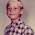Fred and Frank running times puzzle

Here's a puzzle for you to solve.Fred and Frank are two fitness fanatics on a run from A to B. Fred runs half the way and walks the other half. Frank runs for half the time and walks for the other half. They both run and walk at the same speeds. Who finishes first?

Puzzle from Fawn Nguyen's puzzles and brainteasers, set 13

Here's one possible way to solve it. Choose some easy numbers for the distance they travel, for the speeds, and for the time in case of Frank.Then just calculate!

Let's say they run 10 miles per hour and walk 5 miles per hour. Let's also say the distance from A to B is 20 miles.

Fred runs half the way. So, Fred runs 10 miles = 1 h, and he walks 10 miles = 2 h. So he takes a total of 3 hours.

Frank runs half the time and walks half the time. Now, we don't know the time, so let it be t. We can write an equation about the total distance:

(distance running) + (distance walking) = 20 miles

(1/2t) * 10 + (1/2t) * 5 = 20

5t + 2.5t = 20

7.5 t = 20

t = 2 2/3 hours

So, Frank is quicker.